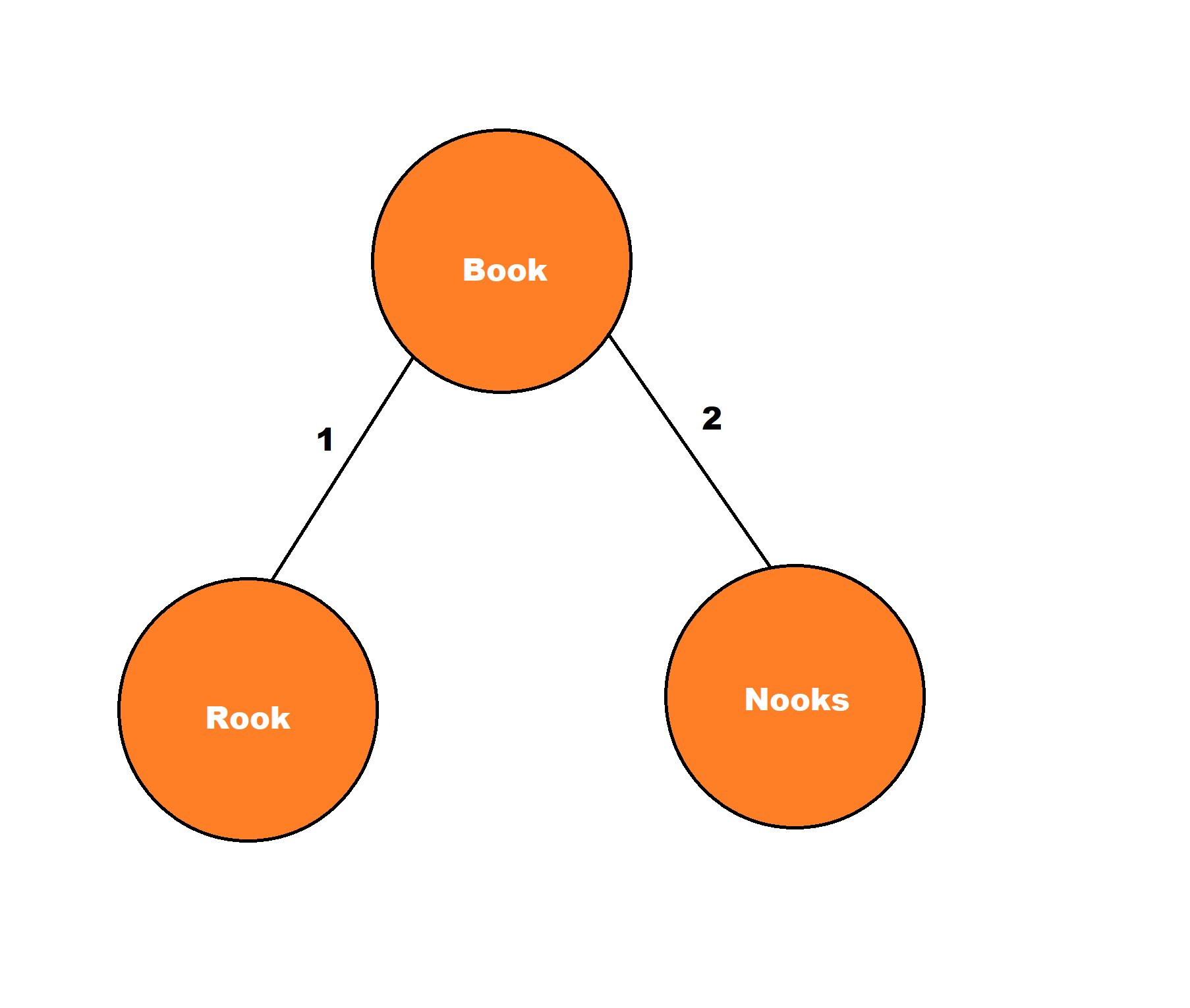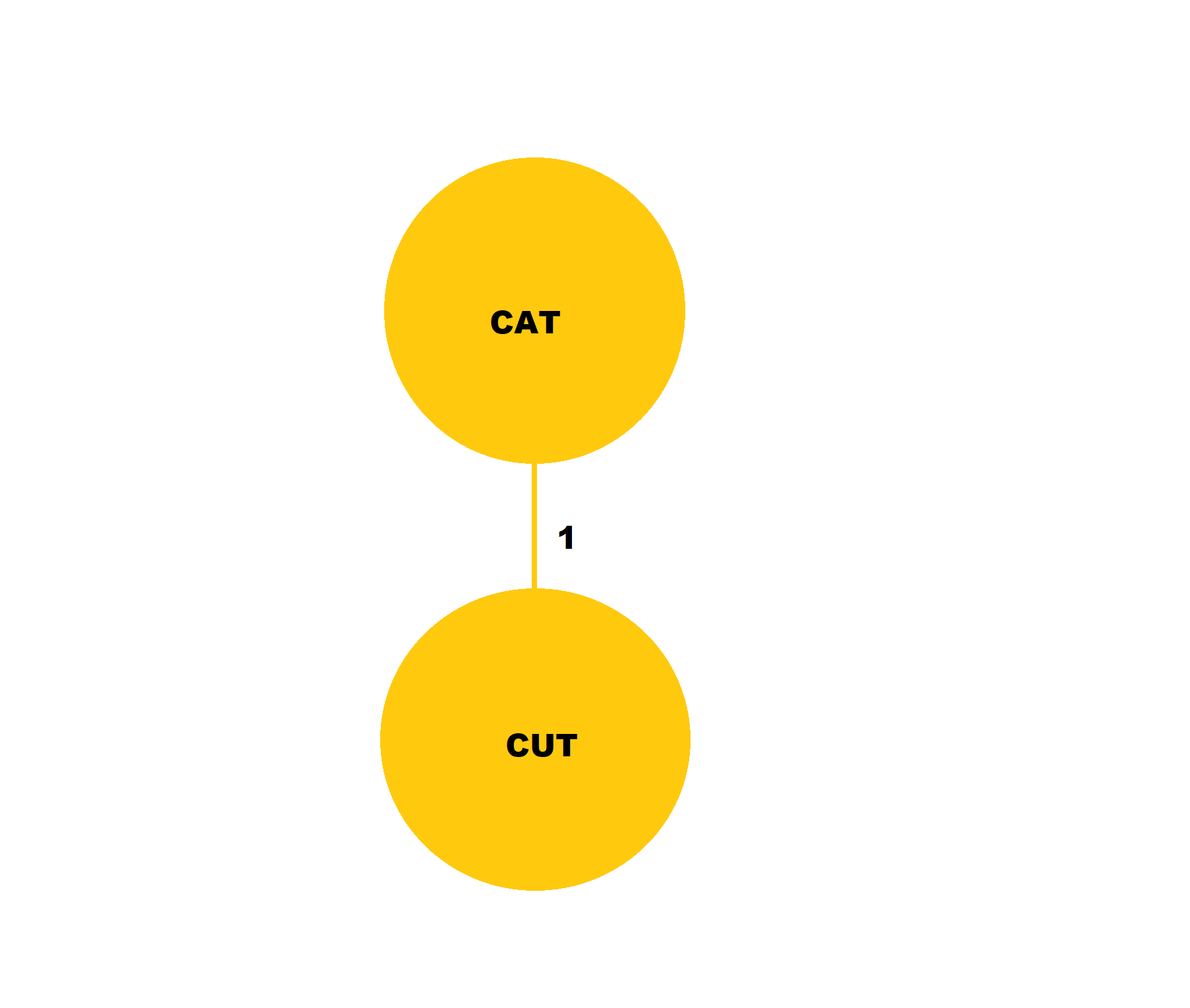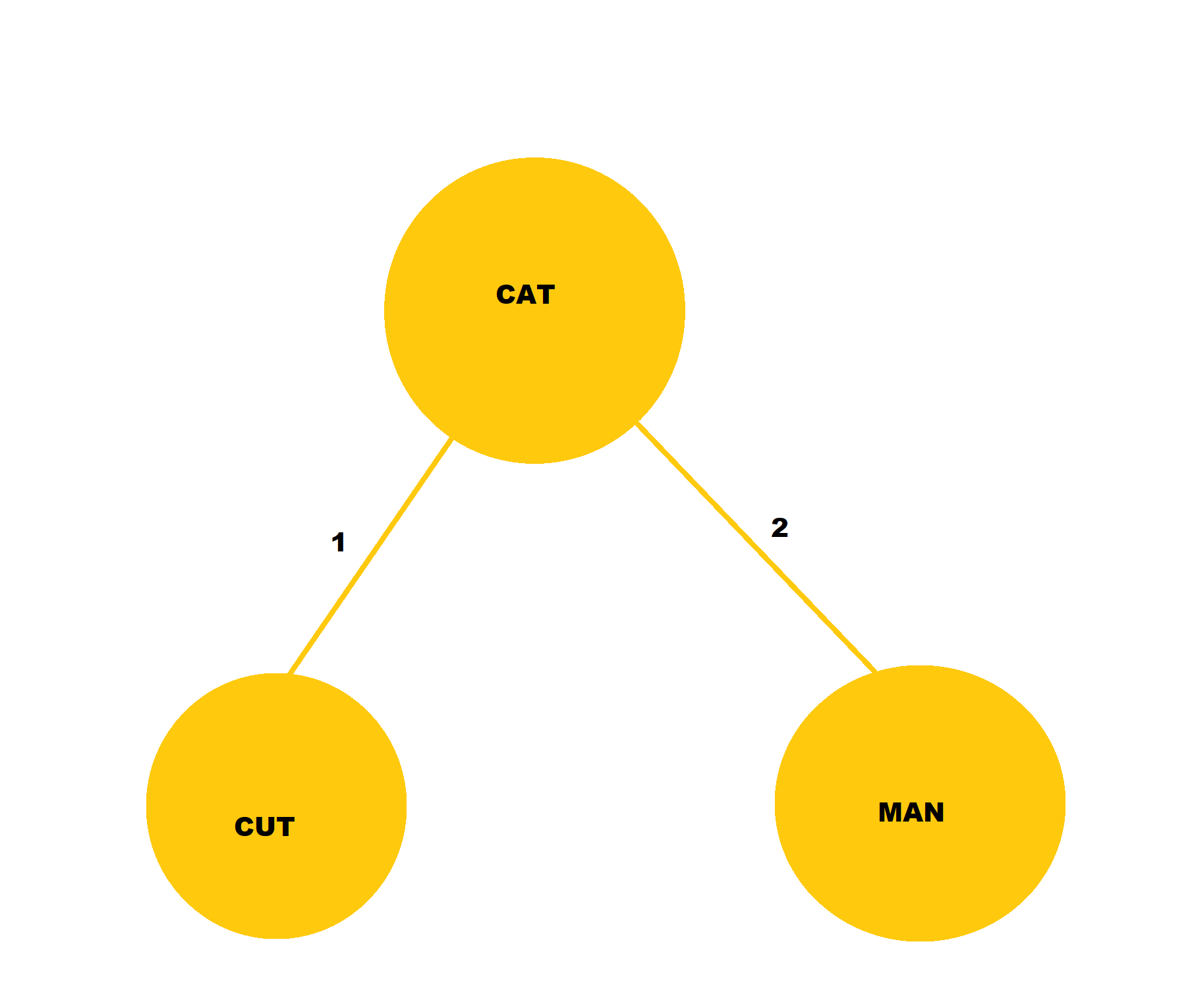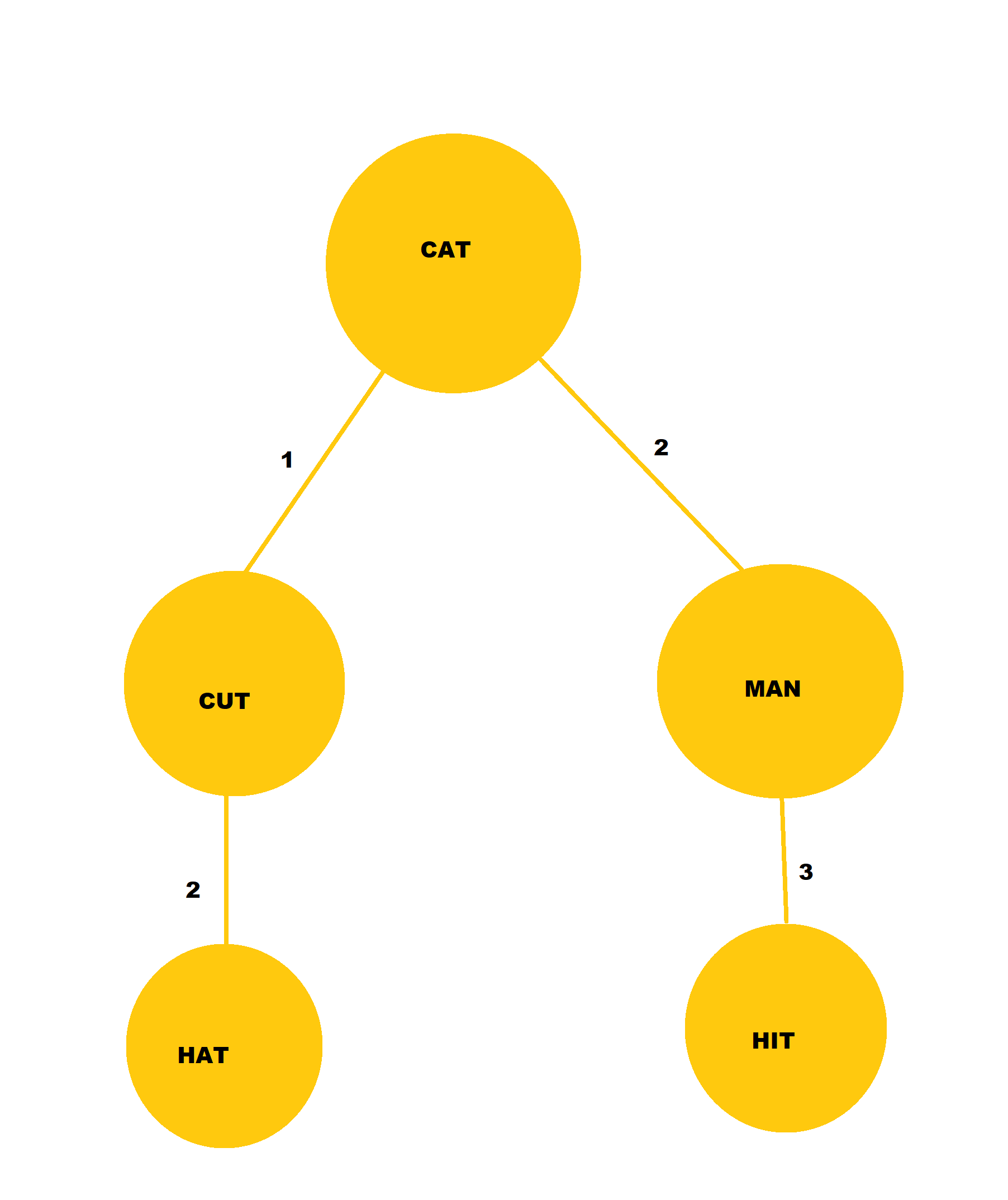×

Search anything:

# Burkhard Keller Tree (BK Tree)

#### Data Structures tree data structureGet this book -> Problems on Array: For Interviews and Competitive Programming

Reading time: 30 minutes | Coding time: 15 minutes

Burkhard Keller Tree or otherwise known as the BK-Tree is a Tree-Based Data Structure that is used to find the near-matches to a String Query. The BK-Trees were first proposed in a paper "Some approaches to best match file searching" by Burkhard and Keller and has been since used as an algorithm for performing Spell Check.

Unlike other algorithms, BK-Tree rests its concepts on the Levenshtein distance which can be understood as the value that is returned after two strings are passed and the minimum number of insertion, deletion and replacements are returned which are required to translate one string into the other. Burkhard Keller Tree are also utilized in implementing the Auto-Complete Feature in many Software and Web Applications and is regarded as more efficient compared to the TRIE and Ternary Search Tree that we have discussed earlier.

In a Burkhard Keller Tree, any arbitrary node can be chosen as the Root Node which can have zero or any number of subtrees. BK Tree is used to perform the Spell Check Operation using the Levenshtein distance which is a string metric that is used to determine the minimum number of single-character edits required to change one word into the another.Levenshtein Distance also forms a Metric Space which is based upon the following three criteria:

• If the distance between X and Y is zero then X is equal to Y.
• The distance from X to Y is equal to the distance between Y to X.
• Triangle Inequality: Path from one point to another must be no longer than any path that goes through any other intermediate path.

In such a case, we will take two queries: one a string while the other one is a value which is the maximum distance, a string can be away from query and still be returned. Now we will take an arbitrary string and compared it to the query which will yield us a resultant distance that satisfies the Triangle Inequality condition.

## Constructing a Burkhard-Keller Tree

BK-Tree is a Tree-Based Data Structure and like any other Tree Data Structure it consists of nodes and edges that connect them. In a BK-Tree, each node consist of the individual words in the dictionary and the number of nodes in a BK-Tree is equal to the number of the words that are being inserted into the dictionary. Each edge that connects the nodes possess an integer value that is corresponsing to the Levenshtein distance.Here we have a simple BK-Tree that has been made taking "Book" as the Root Node while "Rook" and "Nooks" with the edge from Book to Rook marked as 1, since only one replacement is needed to change the string stored at root node to the string stored in left node, while the edge connecting Book to Nooks is marked as 2, since one addition and one replacement is needed to change the string stored at root node to the string stored in the right node.

## Operations on BK-Tree

Like any Tree-based Data Structure, BK-Tree supports various operations like Insertion, Deletion and Search Operations. Other than these, BK-Tree also supports other operations like Autocompletion and Spell Check which we will briefly discuss here.

## 1. Insertion Operation

To construct a BK-Tree we can take any node as the root node and then we have to add some element to the root node, by calculating their Levenshtein Distance and adding them as a left node or the right node, in the same manner as will add nodes in any other Binary Tree. Let's take an array of words ["cat", "cut", "hat", "man", "hit"] and insert them in a BK-Tree:

• We will take "cat" as the root node and insert "cut" to it with the edge integer to be 1 since only 1 replacement is needed to construct "cut" from "cat".• Now we will take "man" and insert it into the BK-Tree. The edge integer will be 2 since two replacements are needed to construct "man" from "cat".• Let's insert ["hat","hit"] in our BK-Tree using the principle of Levenshtein Distance:Here is the Pseudocode of the Insertion Operation in BK-Tree:

``````function insertNode(node){
if(tree is none){
tree=node;
return;
}
current,children=tree; //Assigning the Tree Nodes
while(true){
distance=Distance between current node and new node;
target=children.get(distance);
if target is none{
children[distance]=node;
break;
}
current,children=target;
}
}
``````

## 2. Search Operation

In the Search Operation, our primary aim is to find all the words that are closest given to a query word. This means that we will take an integer 'N' which we will call as the radius. To find any node in the BK-Tree we will just compute the Levenshtein Distance from the query string to the string stored in the root node. If the value that is returned is less than the radius, then the rest of the nodes are recursively processed by selecting all the children of the root node.

Here is the Pseudocode of the Search Operation in a BK-Tree:

``````function search(node,radius){
if(tree is none){
return;
}
candidates=deque(tree) //Extracting elements from Tree
result=[] //Intializing a List for the same
while candidates{
candidate,children=pop();
distance=Distance between Query node and new node;
result.append(distance,candidate);
}
candidates.extend(c for d, c in children.items()
if low <= d <= high)

return result;
}
}
``````

## 3. Deletion operation

There is no true deletion operation in BK Tree which is similar to other trees like Binary Search Tree or Red Black Tree.

To support deletion operation in BK Tree, we shall add a new field in the node named "deleted" which can point to a boolean value true or false.

In case, deleted field is false, the node is considered as a normal node but if it is true, then the node is deleted.

In this case of a deleted node, the node is ignored in the search operation but the insertion operation remains unchanged. We just need to ignore such nodes if encountered.

## Code Implementation

The Code for BK-Tree has been implemented using the Python-3 Programming Language which is an Open-Source General Purpose Programming Language.

``````from collections import deque

class BKTree:
def __init__(self, distance_func):
self._tree = None
self._distance_func = distance_func

if self._tree is None:
self._tree = (node, {})
return

current, children = self._tree
while True:
dist = self._distance_func(node, current)
target = children.get(dist)
if target is None:
children[dist] = (node, {})
break
current, children = target

if self._tree is None:
return []

candidates = deque([self._tree])
result = []
while candidates:
candidate, children = candidates.popleft()
dist = self._distance_func(node, candidate)
result.append((dist, candidate))

candidates.extend(c for d, c in children.items()
if low <= d <= high)
return result
``````

1. Searching a String in a BK-Tree is almost 40 Times faster than a similar Search Operation using Linear Search Operation.
2. Suitable for Nearest Neighbour Search (NNS) with a time complexity of O(log N).
3. Suitable for checking if the word exists in a dictionary and also find possible fixes for misspelled word.
4. Utilizes for boosting performance while implementing Fuzzy Search Operations.
5. Allows to search for the string with fewer comparisons as would need to implement the same in TRIE and Ternary Search Trees.
6. Can be used to implement autocompletion feature#### Harsh Bardhan Mishra

Intern at OpenGenus

Burkhard Keller Tree (BK Tree)